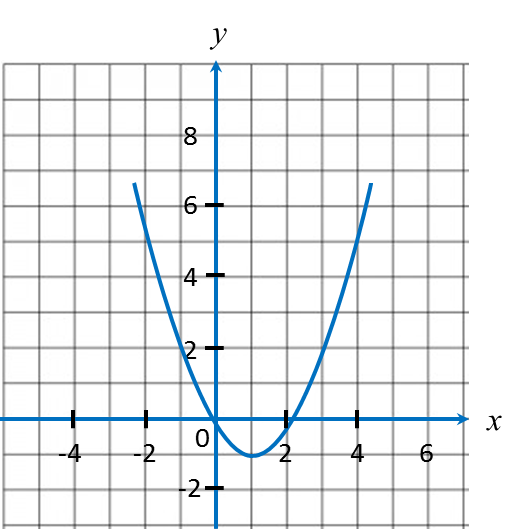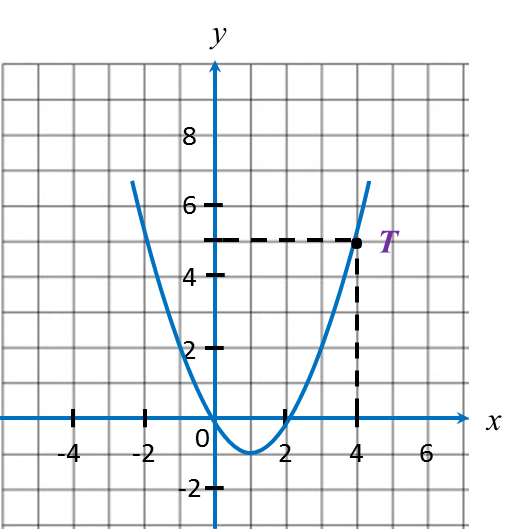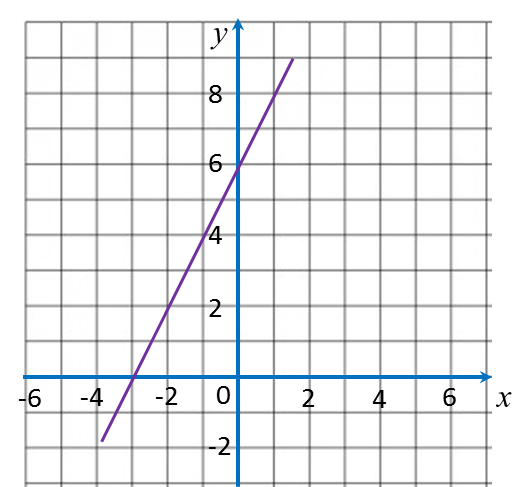# 8.2.1 Graphs of Functions, PT3 Focus Practice

8.2.1 Graphs of Functions, PT3 Focus Practice

Question 1:In the figure shown, when x = 4, the value of y is
Solution:is the point on the graph where x = 4.
Hence, when x = 4, y = 5.

Question 2:
Diagram shows the graph of a function on a Cartesian plane.The equation that represents the function is

Solution:
Use points (0, 6) and (-3, 0) to determine the function.

 Function Value of y when x = 0 x = –3 y = x + 3 3 0 y = x – 3 –3 –6 y = 2x + 6 6 0 (correct) y = 2x – 6 –6 –12

Question 3:
Table below shows the values of variables x and y of a function.

 x –2 0 1 y –6 2 3
Which of the following functions is satisfied by the ordered pair?
A = 7x + 2
B = x3 + 2
C = x + x2 – 2
D = x2 + 2

Solution:
Only y = x3 + 2 is satisfied by all the ordered pairs.
(i) –6 = (–2)3 + 2
(ii)   2 = 03 + 2
(iii)  3 = 13 + 2